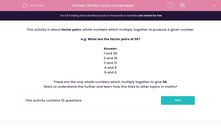# Identify Factors and Multiples

In this worksheet, students select all the factor pairs for a given number.This content is premium and exclusive to EdPlace subscribers.Key stage:  KS 2

Curriculum topic:   Maths and Numerical Reasoning

Curriculum subtopic:   Factors and Multiples

Difficulty level:#### Worksheet Overview

This activity is about factor pairs: whole numbers which multiply together to produce a given number.

e.g. What are the factor pairs of 36?

1 and 36

2 and 18

3 and 12

4 and 9

6 and 6

These are the only whole numbers which multiply together to give 36.

Want to understand this further and learn how this links to other topics in maths?

### What is EdPlace?

We're your National Curriculum aligned online education content provider helping each child succeed in English, maths and science from year 1 to GCSE. With an EdPlace account you’ll be able to track and measure progress, helping each child achieve their best. We build confidence and attainment by personalising each child’s learning at a level that suits them.

Get started